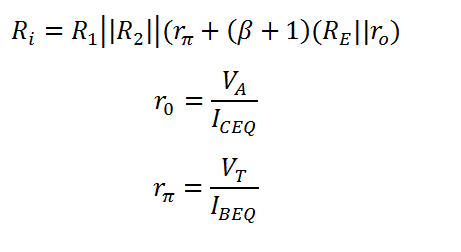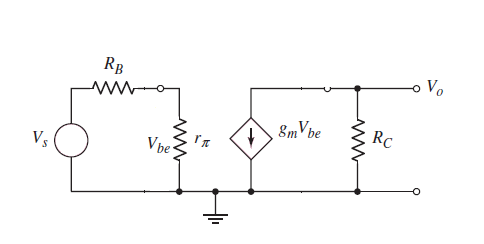# Hybrid Pi Common Emitter BJT Calculator

This calculator computes the Input Resistance (Ri), Small signal output resistance (r0) and Small signal diffusion resistance (rp). The hybrid-pi model is a linearized two-port network approximation to the BJT using the small-signal base-emitter voltage and collector-emitter voltage as independent variables, and the small-signal base current and collector current as dependent variables.

V
mA
K-Ohm
K-Ohm
K-Ohm

#### Result

K-Ohm
K-Ohm
K-Ohm